# Test: Organometallic Chemistry- 2

## 30 Questions MCQ Test Inorganic Chemistry | Test: Organometallic Chemistry- 2

Description
Attempt Test: Organometallic Chemistry- 2 | 30 questions in 90 minutes | Mock test for Chemistry preparation | Free important questions MCQ to study Inorganic Chemistry for Chemistry Exam | Download free PDF with solutions
QUESTION: 1

Solution:
QUESTION: 2

Solution:
QUESTION: 3

### Which one of the following complexes is not expected to exhibit isomerism:

Solution: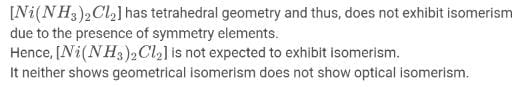QUESTION: 4

The number of metal-metal bonds in [W2(OPh)6] is:

Solution:

No of metal - metal bond= (18n - TVE)/2
Here, TVE = 2(6) + 6(3)

= 30
n = 2 (n denotes the number of metals present)

Hence, No of metal - metal bonds= (36-30)/2

= 3 metal - metal bonds.

QUESTION: 5

Among the following, the correct statement is:

Solution:
QUESTION: 6

Which of the following metal fragments, d9–MLn is isolabal with CH?

Solution:
QUESTION: 7

The cluster Rh6(CO)16, has structure:

Solution:
QUESTION: 8

The cluster having arachno type structure is:

Solution:
QUESTION: 9

Zintl Ion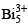is cluster of:

Solution:
QUESTION: 10

Among the following which option is correct about the thermal stability of given compounds:

(P) HMn(CO)5
(Q) HTe(CO)5
(R) HRe(CO)5

Solution:
QUESTION: 11

Which of the following is correct about the basicity:

(P) [Mn(CO)5]
(Q) [Tc(CO)5]
(R) [Re(CO)5]

Solution:
QUESTION: 12

The number of IR bands will be obtain in M(CO)5

Solution:
QUESTION: 13

Nitrosyl ligand binds to d-metal atoms in linear and bent fashion and behaves, respectively, as

Solution:
QUESTION: 14

The correct order of νNO (cm–1) in the following compounds is:

Solution:
QUESTION: 15

If the bond length of CO bond in carbon monoxide is 1.128 Å. Then what is the value of CO bond length in Fe(CO)5:

Solution:
• In Fe(CO)5​ there exists a synergic bond between Fe and CO. Due to synergic bond formation between metal and CO, the bond order of CO decreases.
• As bond order decreases the Bond length increases.Here the only option having greater bond length than 1.128A0 is option A- 1.158A0.
QUESTION: 16

Which of the following in not suitable as catalyst for hydroformylation:

Solution:
QUESTION: 17

The catalyst used for the oxidation of ethylene to acetaldehyde is:

Solution:
QUESTION: 18

Regarding the catalytic cycle of hydrogenation of alkanes involving RhCl(PPh3)3 as t he catalyst, the correct statement is:

Solution:
QUESTION: 19

The catalyst used in the conversion of ethylene to acetaldehyde using Wacker process:

Solution:
QUESTION: 20

The homogeneous catalyst that is used in the hydroformylation or hydrocarbonylation is based on:

Solution:
QUESTION: 21

Metals used in automobile catalytic converters are:

Solution:
QUESTION: 22

Active catalytic species for hydroformylation is:

Solution:
QUESTION: 23

Wikinson’s catalyst:

Solution:
QUESTION: 24

An intermediate formed during the hydroformylation of olefins using Co2(CO)8 as catalyst is:

Solution:
QUESTION: 25

In Monsanto acetic acid process shown below, the role of HI is: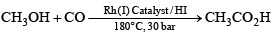Solution:

Explanation : The Monsanto acetic acid process is the major commercial production method for acetic acid. Methanol, which can be generated from synthesis gas ("syn gas", a CO/H2 mixture), is reacted with carbon monoxide in the presence of a catalyst to afford acetic acid. In essence, the reaction can be thought of as the insertion of carbon monoxide into the C-O bond of methanol, i.e. the carbonylation of methanol.

QUESTION: 26

The reaction of acetyl chloride and AlCl3 with ferrocene gives:

Solution:
QUESTION: 27

The infra–red starching frequency vCO of P-S follows the order: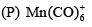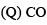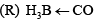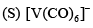Solution:
QUESTION: 28

Ferrocene undergoes Vilsmeir reaction to yield:

Solution:
QUESTION: 29

In hydroformylation process, propene is converted into

Solution:

Hydroformylation, also known as oxo synthesis or oxo process, is an industrial process for the production of aldehydes from alkenes. It is important because aldehydes are easily converted into many secondary products. For example, the resulting aldehydes are hydrogenated to alcohols that are converted to detergents.

When propene reacts with CO and H2 then butanal is formed.

H2 + CO + CH3CH=CH2 → CH3CH2CH2CHO ("normal")

H2 + CO + CH3CH=CH2 → (CH3)2CHCHO ("iso")

Hence B is correct

QUESTION: 30

Which second row transition metal is present in the following compound: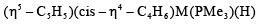Solution:Use Code STAYHOME200 and get INR 200 additional OFF Use Coupon Code